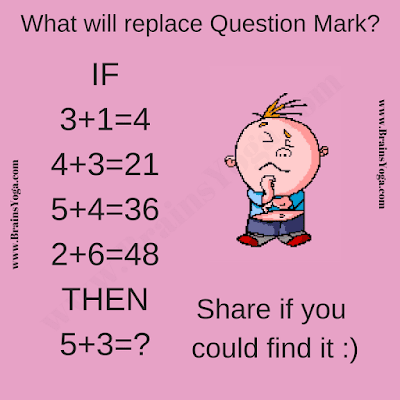## Tuesday, February 18, 2020

This is very interesting logical reasoning equations brain teaser.  Here are some equations which are wrong Mathematically. However, there is some logical connection in these equations which makes these equations correct logically. Can you find the logical reasoning in these equations and then solve the last equation?Can you solve this logical equation?

1.2.